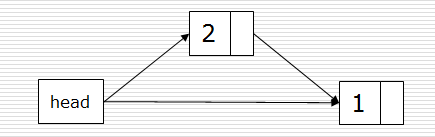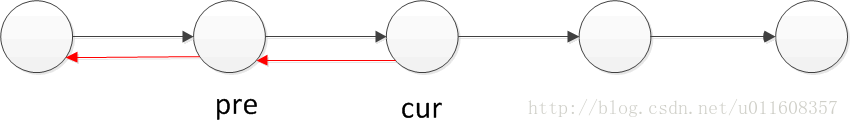Python ┴ą▒Ē┼cµ£▒ĒĄ─ģ^äeįöĮŌ

Ė³ą┬ĢrķgŻ║2021─Ļ09į┬10╚š 10:15:57   ū„š▀Ż║Yake1965

python ┴ą▒Ē║═µ£▒ĒĄ─ģ^äe

class Node:
def __init__(self, value, next=None):
self.value = value
self.next = next

ĮėŽ┬üĒŻ¼╬ęéāŠ═┐╔ęįīó╦∙ėąĄ─╣Ø³cśŗįņ│╔ę╗éĆ┴ą▒Ē┴╦Ż║

>>> L = Node("a", Node("b", Node("c", Node("d"))))
>>> L.next.next.value
'c'

▀@╩Ūę╗éĆ╦∙ų^Ą─å╬Ž“µ£▒ĒŻ¼ļpŽ“µ£▒ĒĄ─Ė„╣Ø³cųą▀ĆąĶę¬│ųėąę╗éĆųĖŽ“Ū░ę╗éĆ╣Ø³cĄ─ę²ė├

Ą½╩ŪŠ▀¾wĄĮ insert ▓┘ū„╔ŽŻ¼ŪķørėųĢ■ėą╦∙▓╗═¼ĪŻī”ė┌µ£▒ĒČ°čįŻ¼ų╗ę¬ų¬Ą└┴╦ę¬į┌──└’ł╠ąą insert ▓┘ū„Ż¼Ųõ▓┘ū„│╔▒Š╩ŪĘŪ│ŻĄ═Ą─Ż¼¤ošōįō┴ą▒ĒųąėąČÓ╔┘į¬╦žŻ¼Ųõ▓┘ū„Ģrķg┤¾ų┬╔Ž╩ŪŽÓ═¼Ą─ĪŻČ°öĄĮMŠ═▓╗ę╗śė┴╦Ż¼╦³├┐┤╬ł╠ąą insert ▓┘ū„Č╝ąĶę¬ęŲäė▓Õ╚ļ³cėę▀ģĄ─╦∙ėąį¬╦žŻ¼╔§ų┴į┌▒žę¬Ą─Ģr║“Ż¼╬ęéā┐╔─▄▀ĆąĶę¬īó▀@ą®┴ą▒Ēį¬╦žš¹¾w░ßĄĮę╗éĆĖ³┤¾Ą─öĄĮMųą╚źĪŻ

1.öĄĮMĄ─į¬╦ž╩Ū┤µĘ┼į┌ŚŻųąĄ─Ż¼µ£▒ĒĄ─į¬╦žį┌Ččųą
2.ūx╚Ī▓┘ū„Ż║öĄĮMĢrķgÅ═ļsČ╚×ķO(1)Ż¼µ£▒Ē×ķO(n)
3.▓Õ╚ļ╗“äh│²▓┘ū„Ż║öĄō■ĢrķgÅ═ļsČ╚×ķO(n)Ż¼µ£▒Ē×ķO(1)

┴ą▒Ē
ĻPė┌┴ą▒ĒĄ─┤µā”Ż║

┴ą▒Ēķ_▒┘Ą─ā╚┤µ┐šķg╩Ūę╗ēK▀B└mĄ─ā╚┤µŻ¼░č▀@éĆā╚┤µĄ╚Ęų│╔ÄūĘ▌(å╬╬╗╩Ūūų╣Ø)Ż¼╦¹╩Ū▀B└m┤µā”Ą─ĪŻ
╚ń╣¹ę╗éĆ┴ą▒ĒķLČ╚ęčØMŻ¼į┘append╠Ē╝ėį¬╦žĄ─įÆŻ¼Ģ■į┌ā╚┤µųąųžą┬ķ_▒┘ę╗éĆ2▒ČĄ─ā╚┤µ┐šķgęį┤µā”ą┬į¬╦žŻ¼įŁ┴ą▒Ēā╚┤µĢ■▒╗ŪÕ│²ĪŻ

┴ą▒Ē┼cµ£▒ĒÅ═ļsČ╚Ż║

░┤į¬╦žųĄ▓ķšę:
░┤Ēśą“▓ķšęŻ¼Å═ļsČ╚╩Ūę╗śėĄ─ĪŻ
░┤Ž┬ś╦▓ķšę:
┴ą▒Ē╩ŪO( 1 )
µ£▒Ē╩ŪO(n)
į┌─│į¬╦ž║¾▓Õ╚ļ:
┴ą▒Ē╩ŪO(n)
µ£▒Ē╩ŪO( 1 )
äh│²─│į¬╦ž:
┴ą▒Ē╩ŪO(n)
µ£▒Ē╩ŪO( 1 )

µ£▒Ē------->┴ą▒ĒŽÓī”æ¬Ą─öĄō■ĮYśŗ

class  Node( object ):
ĪĪĪĪ def  __init__( self ,item):
self .item  =  item  # öĄō■ė“
self . next  =  None  # ųĖßśė“

n1  =  Node( 1 )
n2  =  Node( 2 )
n3  =  Node( 3 )

n1. next  =  n2
n2. next  =  n3
print (n1. next . next .item)

2Īóµ£▒Ē▒ķÜvŻ║(Ņ^╣Ø³cĄ─ūāäė)class  Node( object ):
ĪĪĪĪ def  __init__( self ,item):
self .item  =  item  # öĄō■ė“
self . next  =  None  # ųĖßśė“

n1  =  Node( 1 )
n2  =  Node( 2 )
n3  =  Node( 3 )

n1. next  =  n2
n2. next  =  n3
print (n1. next . next .item)

3Īóµ£▒Ē╣Ø³cĄ─▓Õ╚ļ║═äh│²▓┘ū„(ĘŪ│ŻĘĮ▒ŃŻ¼ĢrķgÅ═ļsČ╚Ą═)

▓Õ╚ļŻ║p  =  Node( 5 )  # ę¬▓Õ╚ļĄ─ųĄ
curNode  =  Node( 1 )  # ś╦ųŠ╬╗
# Ēśą“▓╗─▄üyŻ¼Ę±ätŠ═šę▓╗ĄĮįŁµ£▒ĒųąĄ─Ž┬ę╗éĆųĄ
p. next  =  curNode. next  # ųĖČ©▓Õ╚ļųĄų«║¾Ą─ųĄ×ķś╦ųŠ╬╗ų«║¾Ą─ųĄ
curNode. next  =  p   # ╚╗║¾į┘░čįŁŽ╚Ą─µ£nextųĖŽ“Ė─│╔▓Õ╚ļĄ─ųĄ

äh│²Ż║curNode ┤·▒Ē«öŪ░ųĄ
p  =  curNode. next  # ▒Ē╩Šę¬äh│²Ą─öĄ
del  p äh│²öĄĪĪĪĪl  =  Node()  # ╩╝ĮKųĖŽ“Ņ^╣Ø³c
ĪĪĪĪ for  num  in  li:
s  =  Node(num)
s. next  =  l. next
l. next  =  s
ĪĪĪĪ return  lĪĪĪĪl  =  Node()
ĪĪĪĪr  =  l  # rųĖŽ“╬▓╣Ø³c
ĪĪĪĪ for  num  in  li:
s  =  Node(num):
r. next  =  s

ļpµ£▒Ē
ļpµ£▒Ēųą├┐éĆ╣Ø³cėąā╔éĆųĖßśŻ║ę╗éĆųĖŽ“║¾├µ╣Ø³cŻ¼ę╗éĆųĖŽ“Ū░├µ╣Ø³cĪŻclass  Node( object ):
ĪĪĪĪ def  __init__( self ,item = None ):
self .item  =  item    # ėøõø«öŪ░ųĄ
self . next  =  None    # ėøõøŽ┬ę╗éĆųĄ
self .prior  =  None   # ėøõøŪ░ų├Ą─ę╗éĆųĄ

2Īóļpµ£▒Ē╣Ø³cĄ─▓Õ╚ļ║═äh│²curNode  =  Node( 1 )  # ╚Īę╗öĄō■ū„×ķś╦ųŠ╬╗

1Ż®▓Õ╚ļŻ║

p  =  Node( 2 )  # ę¬▓Õ╚ļĄ─öĄ
p. next  =  curNode. next  # ųĖČ©▓Õ╚ļöĄĄ─next ╩Ū «öŪ░öĄĄ─next
curNode. next .prior  =  p  # ųĖČ©▓Õ╚ļöĄĄ─Ž┬ę╗éĆöĄĄ─ Ū░ų├öĄ×ķ«öŪ░Ą─öĄųĄ
p.prior  =  curNode  # ▓Õ╚ļöĄĄ─Ū░ų├öĄ×ķ ś╦ųŠ╬╗
curNode. next  =  p  # ųĖČ©Ż¼ś╦ųŠ╬╗Ą─nextöĄ╩Ū«öŪ░ę¬▓Õ╚ļĄ─öĄ

2Ż®äh│²Ż║

p  =  curNode. next  # ś╦ųŠ╬╗Ą─Ž┬ę╗éĆöĄŻ¼ę¬äh│²Ą─öĄ
curNode. next  =  p. next  # īónextųĖŽ“Ž┬ę╗éĆöĄ
p. next .prior  =  curNode # īóę¬äh│²öĄĄ─Ž┬ę╗éĆöĄĄ─Ū░ų├öĄĖ─×ķś╦ųŠ╬╗
del  p  # äh│²«öŪ░öĄ

ĪĪĪĪl  =  Node()
ĪĪĪĪr  =  l
ĪĪĪĪ for  num  in  li:
s  =  Node(num)
r. next  =  s
s.prior  =  r
r  =  s
ĪĪĪĪ return  l,r

å╬µ£▒Ē─µų├class  Node( object ):

def  __init__( self ,item = None , next = None ):
self .item  =  item  # öĄō■ė“
self . next  =  next  # ųĖßśė“

cur  =  link. next  # ėøõøŽ┬ę╗╣Ø³cĄ─ųĄ
pre. next  =  None  # Ž╚īó«öŪ░╣Ø³cĄ─nextųĖŽ“Č©×ķNone

while  cur:  # µ£▒Ēųąę╗ų▒ėąųĄ
tmp  =  cur. next  # ½@╚ĪcurĄ─Ž┬ę╗éĆųĄŻ¼┼RĢr┘xųĄĮotmp
cur. next  =  pre  # īócurųĄųĖŽ“pre
cur  =  tmp

return  pre  # ░č«öŪ░ųĄĘĄ╗ž

#æ¬ė├
link  =  Node( 1 , Node( 2 , Node( 3 , Node( 4 , Node( 5 , Node( 6 , Node( 7 , Node( 8 , Node( 9 )))))))))
while  r:
print ( "{0}---->" . format (r.item))  # ▌ö│÷─µų├║¾Ą─«öŪ░ųĄ
r  =  r. next  # ½@╚ĪŽ┬ę╗éĆŻ¼ųžą┬┘xĮorŻ¼╚╗║¾Į╗Įo╔Ž▀ģ▌ö│÷

┴ą▒ĒĄ─īŹ¼FÖCųŲ

┴ą▒ĒĄ─īŹ¼F┐╔ęį╩ŪöĄĮM╗“š▀µ£▒ĒĪŻ┴ą▒Ē╩Ūę╗ĘNĒśą“▒ĒŻ¼Ēśą“▒Ēę╗░Ń╩ŪöĄĮMĪŻ┴ą▒Ē╩Ūę╗éĆŠĆąį▒ĒŻ¼╦³į╩įSė├æ¶į┌╚╬║╬╬╗ų├▀Mąą▓Õ╚ļĪóäh│²ĪóįLå¢║═╠µōQį¬╦žĪŻ

┴ą▒ĒĄ─īŹ¼F╩Ū╗∙ė┌öĄĮM╗“š▀╗∙ė┌µ£▒ĒĮYśŗŻ¼«ö╩╣ė├┴ą▒ĒĄ³┤·Ų„Ą─Ģr║“Ż¼ļpŽ“µ£▒ĒĮYśŗ▒╚å╬µ£▒ĒĮYśŗĖ³┐ņĪŻ
Python ųąĄ─┴ą▒Ēėó╬─├¹╩Ū listŻ¼ę“┤╦║▄╚▌ęū┼c C šZčįųąĄ─µ£▒ĒĖŃ╗ņ┴╦Ż¼ę“×ķį┌ C šZčįųą┤¾╝ęĮø│ŻĮoµ£▒Ē├³├¹×ķ listĪŻ╩┬īŹ╔Ž CPythonŻ¼ę▓╩Ū╬ęéā│ŻęŖĄ─ė├ C šZčįķ_░lĄ─ Python ĮŌßīŲ„Ż¼Python šZčįĄūīė╩Ū C šZčįīŹ¼FĄ─Ż®ųąĄ─┴ą▒ĒĖ∙▒Š▓╗╩Ū┴ą▒ĒĪŻį┌ CPython ųą┴ą▒Ē▒╗īŹ¼F×ķķLČ╚┐╔ūāĄ─öĄĮMĪŻ

┐éĄ─üĒšfŻ¼PythonųąĄ─┴ą▒Ē╩Ūę╗éĆäėæBĄ─Ēśą“▒ĒŻ¼Č°Ēśą“▒Ē┤¾ČÓ╩Ūė╔öĄĮMīŹ¼FĄ─ĪŻ

µ£▒Ē

class Node(object):
"""╣Ø³c"""
def __init__(self, elem):
self.elem = elem
self.next = None

µ£▒ĒĘŌčbĄ─ę╗ŽĄ┴ą▓┘ū„Ż║

"""å╬µ£▒Ē"""
def __init__(self, node=None):

def is_empty(self):
"""µ£▒Ē╩ŪĘ±×ķ┐š"""

def length(self):
"""µ£▒ĒķLČ╚"""
# curė╬ś╦Ż¼ė├üĒęŲäė▒ķÜv╣Ø³c
# countėøõøöĄ┴┐
count = 0
while cur != None:
count += 1
cur = cur.next
return count

def travel(self):
"""▒ķÜvš¹éĆµ£▒Ē"""
while cur != None:
print(cur.elem, end=" ")
cur = cur.next
print("")

node = Node(item)

def append(self, item):
node = Node(item)
if self.is_empty():
else:
while cur.next != None:
cur = cur.next
cur.next = node

def insert(self, pos, item):
:param  pos Å─0ķ_╩╝
"""
if pos <= 0:
elif pos > (self.length()-1):
self.append(item)
else:
count = 0
while count < (pos-1):
count += 1
pre = pre.next
# «öčŁŁh═╦│÷║¾Ż¼preųĖŽ“pos-1╬╗ų├
node = Node(item)
node.next = pre.next
pre.next = node

def remove(self, item):
"""äh│²╣Ø³c"""
pre = None
while cur != None:
if cur.elem == item:
# Ž╚┼ąöÓ┤╦ĮY³c╩ŪĘ±╩ŪŅ^╣Ø³c
# Ņ^╣Ø³c
else:
pre.next = cur.next
break
else:
pre = cur
cur = cur.next

def search(self, item):
"""▓ķšę╣Ø³c╩ŪĘ±┤µį┌"""
while cur != None:
if cur.elem == item:
return True
else:
cur = cur.next
return False

µ£▒Ē┼c┴ą▒ĒĄ─▓Ņ«É

µ£▒ĒŻ║┤µā”ĄžųĘĘų╔óĄ─Ż¼ąĶę¬Ī░ųĖßśĪ▒ųĖŽ“Ą─ŠĆąį▒Ē

┴ą▒Ē╩Ūę╗éĆŠĆąįĄ─╝»║ŽŻ¼╦³į╩įSė├æ¶į┌╚╬║╬╬╗ų├▓Õ╚ļĪóäh│²ĪóįLå¢║═╠µōQį¬╦žĪŻ
┴ą▒ĒīŹ¼F╩Ū╗∙ė┌öĄĮM╗“╗∙ė┌µ£▒ĒĮYśŗĄ─ĪŻ«ö╩╣ė├┴ą▒ĒĄ³┤·Ų„Ą─Ģr║“Ż¼ļpµ£▒ĒĮYśŗ▒╚å╬µ£▒ĒĮYśŗĖ³┐ņĪŻ
ėąą“Ą─┴ą▒Ē╩Ūį¬╦ž┐é╩Ū░┤šš╔²ą“╗“š▀ĮĄą“┼┼┴ąĄ─į¬╦žĪŻ

īŹ¼F╝Ü╣Ø

└¹ė├ list.pop(i) ╗“ list.remove(value) äh│²ę╗éĆį¬╦žĪ¬Ī¬Å═ļsČ╚O(N)

┐╔ęį▓╔ė├ĢrķgÅ═ļsČ╚üĒ║Ō┴┐Ż║

index() O(1)
append O(1)
pop() O(1)
pop(i) O(n)
insert(i,item) O(n)
del operator O(n)
iteration O(n)
contains(in) O(n)
get slice[x:y] O(k)
del slice O(n)
set slice O(n+k)
reverse O(n)
concatenate O(k)
sort O(nlogn)
multiply O(nk)